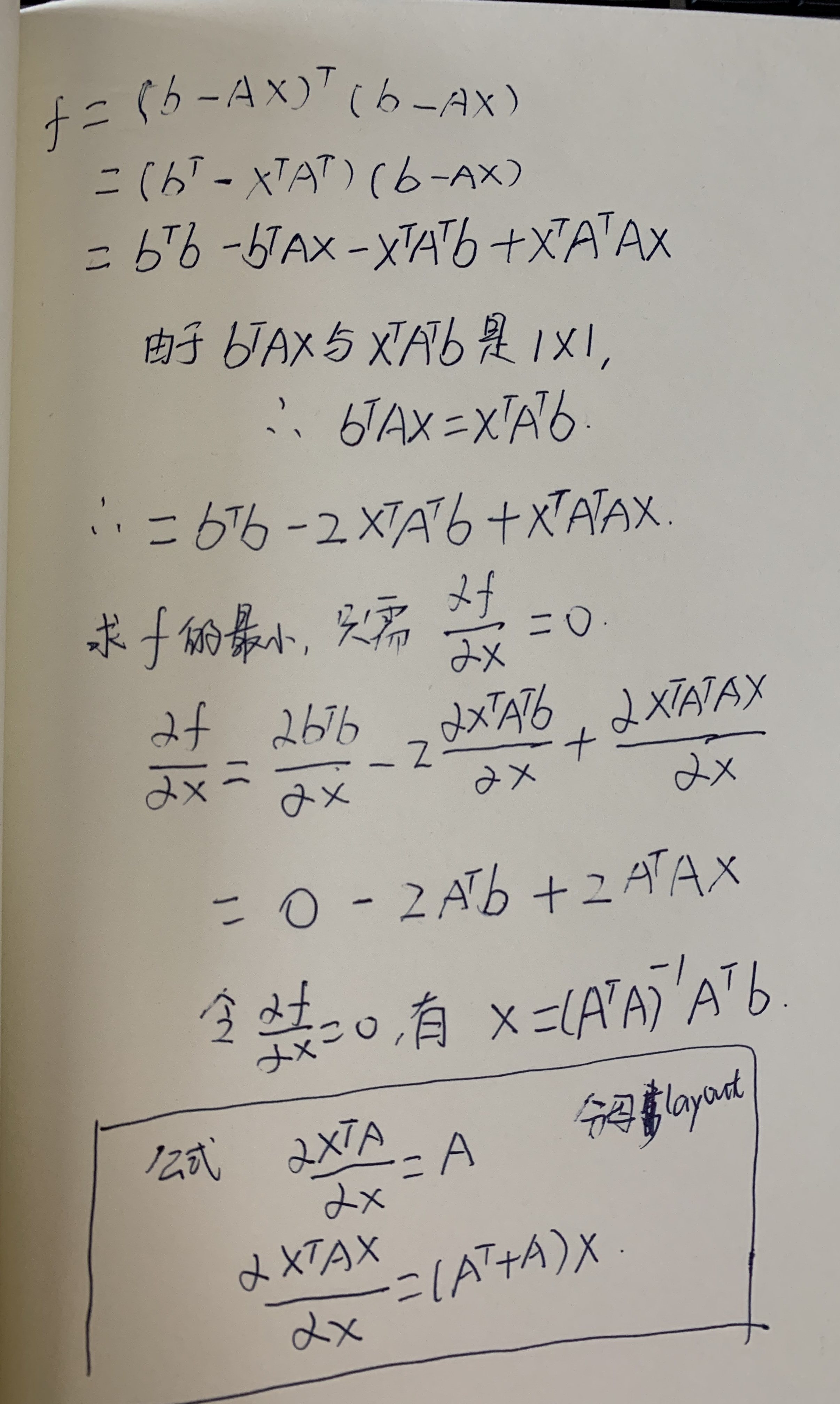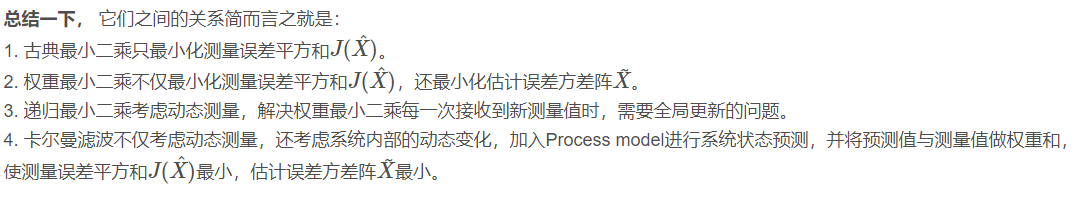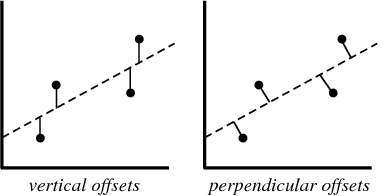• 最小二乘、加权最小二乘、迭代加权最小二乘（迭代重加全最小二乘最小二乘： 最小二乘法（又称最小平方法）是一种数学优化技术。它通过最小化误差的平方和寻找数据的最佳函数匹配。利用最小二乘法可以简便地...
最小二乘、加权最小二乘（WLS）、迭代加权最小二乘（迭代重加全最小二乘）（IRLS）

最小二乘：

最小二乘法（又称最小平方法）是一种数学优化技术。它通过最小化误差的平方和寻找数据的最佳函数匹配。利用最小二乘法可以简便地求得未知的数据，并使得这些求得的数据与实际数据之间误差的平方和为最小。最小二乘法还可用于曲线拟合。

$X=\begin{bmatrix} x_{11} & x_{12} & ... \\ x_{21} & x_{22} & ... \\ x_{31} & x_{32} & ... \\ ... & ... & x_{mn} \end{bmatrix}$     $Y=\begin{bmatrix} y_{1}\\ ...\\ y_{m} \end{bmatrix}$  $\mathbf{\theta}=\begin{bmatrix} \theta_{1}\\ ...\\ \theta_{n} \end{bmatrix}$
$X$中每一列为特征，每一行代表一个样本；$Y$为标签；$\theta$是参数
有优化问题：$f(\theta)=\frac{1}{2}\left | \left | X\theta-Y \right | \right |^{2}$
$f(\mathbf{\theta})=\frac{1}{2}(\mathbf{\theta}^{T}X^{T}X\mathbf{\theta}-\mathbf{\theta}^{T}X^{T}Y-Y^{T}X\mathbf{\theta}+Y^{T}Y)$
对函数$f(\theta)$关于参数$\mathbf{\theta}$求偏导：$\frac{\partial f(\mathbf{\theta}))}{\partial \mathbf{\theta}}=X^{T}X\mathbf{\theta}-X^{T}Y=0$
$\mathbf{\theta}=(X^{T}X)^{-1}X^{T}Y$
得到$\mathbf{\theta}$的解析解。

加权最小二乘（WLS）：
加权最小二乘是在普通最小二乘的基础上对每个样本引入权重，调整不同样本误差对损失函数的贡献率。

加权最小二乘法是对原模型进行加权，使之成为一个新的不存在异方差性的模型，然后采用普通最小二乘法估计其参数的一种数学优化技术。
异方差性是相对于同方差而言的。所谓同方差，是为了保证回归参数估计量具有良好的统计性质，经典线性回归模型的一个重要假定：总体回归函数中的随机误差项满足同方差性，即它们都有相同的方差。

$X$、$Y$、$\theta$定义如上，引入对角矩阵$W$
$W=\begin{bmatrix} w_{11} & ... &0 & 0\\ 0 & w_{22} &... &0 \\ ...& ... & ... &... \\ 0& 0& ... & w_{mm} \end{bmatrix}$
损失函数变为：$f(\mathbf{\theta})=\frac{1}{2}\left \| W(X\mathbf{\theta}-Y) \right \|^{2}$
$f(\mathbf{\theta})=\frac{1}{2}(W\mathbf{\theta}^{T}X^{T}A\mathbf{\theta}-W\mathbf{\theta}^{T}A^{T}Y-WY^{T}A\mathbf{\theta}+WY^{T}Y)$
对函数$f(\theta)$关于参数$\mathbf{\theta}$求偏导：$\frac{\partial f(\mathbf{\theta})}{\partial \mathbf{\theta}}=-X^{T}W^{T}WY+X^{T}W^{T}WX\mathbf{\theta}=0$
$\ \mathbf{\theta}=(X^{T}W^{T}WX)^{-1}X^{T}W^{T}WY$

迭代重加权最小二乘（IRLS）：

迭代重加权最小二乘（IRLS）与迭代加权最小二乘（IWLS）虽然名字不一样，但是内容确实一样，IRLS与IWLS指的都是同一种算法，（我在网上查了很多博客，发现许多人喜欢把IRLS叫做迭代加权最小二乘）
IRLS用来求解p范数的问题，将p范数问题转化为2范数求解。

目标函数：$f(\mathbf{\theta})=\left \| AX-Y \right \|_{p}^{p}$，这里的$\left \| AX-Y \right \|_{p}$是一个$p$范数。计算方式如：$\left \| X \right \|_{p}=\sqrt[p]{x_{1}^{p}+x_{2}^{p}+...+x_{m}^{p}}$。
所以$f(\mathbf{\theta})=\underset{\mathbf{\theta}}{arg\min}\sum_{i=1}^{m}(x_{i}\mathbf{\theta}-y_{i})^{p}$
$f(\mathbf{\theta})=\underset{\mathbf{\theta}}{arg\min}\sum_{i=1}^{m}(x_{i}\mathbf{\theta}-y_{i})^{p-2}(x_{i}\mathbf{\theta}-y_{i})^{2}$
$f(\mathbf{\theta})=\underset{\mathbf{\theta}}{arg\min}\sum_{i=1}^{m}w_{i}^{2}(x_{i}\mathbf{\theta}-y_{i})^{2}$
所以：
$w_{i}^{2}=|x_{i}\mathbf{\theta}-y_{i}|^{p-2}$
$w_{i}=|x_{i}\mathbf{\theta}-y_{i}|^{\frac{p-2}{2}}$
写成矩阵形式就是：
$f(\mathbf{\theta})=\frac{1}{2}||W(X\mathbf{\theta}-Y)||^{2}=\frac{1}{2}W^{2}\left \| X\mathbf{\theta}-Y \right \|^{2}$
$\mathbf{\theta}_{new}=(X^{T}W^{T}_{new}W_{new}X)^{-1}X^{T}W^{T}_{new}W_{new}Y$
$W_{new}=|X\mathbf{\theta}_{old}-Y|^{\frac{p-2}{2}}$
IRLS参考文献：Burrus C S. Iterative re-weighted least squares[J]. Comm.pure Appl.math, 2009, 44(6):1-9.


展开全文算法
•研究论文
• 一： 线性最小二乘 线性最小二乘可以用来求模型的拟合等，例如，直线，平面拟合等。但总归说来模型方程必须是线性的。例如直线拟合中 直线为为了模拟该直线，我们采样了n组点（比如100组），分别记录了他们的坐标...
一： 线性最小二乘

线性最小二乘可以用来求模型的拟合等，例如，直线，平面拟合等。但总归说来模型方程必须是线性的。例如直线拟合中

直线为 为了模拟该直线，我们采样了n组点（比如100组），分别记录了他们的坐标。我们知道该直线方程只需要三组点就可以解出参数。但是由于噪声的存在，我们有100组点，不能随意的取三组，然后解方程。那么最好的办法就是最小二乘解。

假设我们已经知道直线参数a', b'。对于方程y = ax + b ,现在未知数是a和b，所以我们将方程写成矩阵的形式如下：

, 由于这里x, y是已知数，所以我们转换下未知数的表达，我们用向量X 表示未知数a, b。用向量A 表示系数矩阵，用未知数b表示观测值y的向量。

则方程为 AX = b; A 是100x2 矩阵，X 是2x1列向量，b是100x1列向量。定义误差为 e = b - AX,所以最优的参数值应该是符合以下公式展开全文• 古典最小二乘估计，加权最小二乘估计，递归最小二乘估计以及卡尔曼滤波 古典最小二乘估计，以测量误差的平方和作为损失函数。 根据高斯-马尔可夫定理，古典最小二乘估计在线性测量系统中，在测量噪声为零均值，同方...
古典最小二乘估计，加权最小二乘估计，递归最小二乘估计以及卡尔曼滤波
古典最小二乘估计，以测量误差的平方和作为损失函数。
根据高斯-马尔可夫定理，古典最小二乘估计在线性测量系统中，在测量噪声为零均值，同方差和独立的情况下，是最佳线性无偏估计（Best linear unbias estimator - BLUE），其中最佳指的是最小二乘估计值的方差与其他估计的方差相比最小。
而加权最小二乘估计，就是在损失函数中加上权重W，用于量化不同测量对于结果的影响程度。
当测量噪音不为同方差时，古典最小二乘法则不为BLUE，因为估计方差并不是最小了。而当W为R(测量噪音协方差矩阵)的逆时，解决了这个问题，加权最小二乘估计变成了BLUE。
而递归最小二乘法即解决了加权最小二乘估计每次计算都需要批处理的问题，做到了实时测量，提高了效率。
而卡尔曼滤波就是在递归最小二乘的基础上加上了系统内部的动态变化。当然这是卡尔曼滤波关于RLS的思路，还可以从高斯分布角度去思考，但目前不知道如何将这两者联合起来…参考资料：
笔记本！
高斯-马尔可夫定理
卡尔曼滤波与最小二乘法
卡尔曼滤波-高斯分布


展开全文卡尔曼滤波算法 自动驾驶
• // 为终止条件设置最小转换差异 icp.setMaxCorrespondenceDistance(10); // 设置对应点对之间的最大距离（此值对配准结果影响较大）。 icp.setEuclideanFitnessEpsilon(0.001); // 设置收敛条件是均方误差和小于...shell
• 数据拟合，最小二乘，正交最小二乘，卡尔曼拟合加权最小方差拟合全部可以运行matlab
• 通常意义上的最小二乘可以参考下面的文章： https://blog.csdn.net/qq_16587307/article/details/81317253 http://mathworld.wolfram.com/LeastSquaresFitting.html 通常意义的最小二乘参考下面的图片的左图...
通常意义上的最小二乘可以参考下面的文章：

https://blog.csdn.net/qq_16587307/article/details/81317253

http://mathworld.wolfram.com/LeastSquaresFitting.html

通常意义的最小二乘参考下面的图片的左图(vertical offsets)：

在二维平面下如果要进行线性拟合，要找到一条直线，使得这些离散的点到这条直线的竖直偏差的平方和最小。

这是经典的最小二乘法，也是被大力推广的最小二乘法，也是强烈建议使用的方法。今天讨论另外一种最小二乘（上图perpendicular offsets）：

在二维平面下如果要进行线性拟合，要找到一条直线，使得这些离散的点到这条直线的垂直偏差的平方和最小。

求一个点到一条直线的距离，很容易搜的到，这里不做证明，直接给出结论：

设直线 L 的方程为Ax+By+C=0，点 P 的坐标为（Xo，Yo），则点 P 到直线 L 的距离为：

这种不是常用的perpendicular offsets的最小二乘法，它的参考文献如下，给出了非常详细的推导过程：

http://mathworld.wolfram.com/LeastSquaresFittingPerpendicularOffsets.html

不过，这篇文章的公式(16）可以进一步等价为下面的式子:

系数b就可以通过上式求得。其中表示的方差，表示的方差，表示，的协方差。

定义,因此根据一元二次方程根的求解方法得到(与vertical offsets是不一样的)：

我们可以发现这种最小二乘法方式下的系数a求出来的结果和vertical offsets结果是一样的：

文献说参数b与vertical offsets的显得很笨拙，且参数维度增加无法进一步拓展。而传统的vertical offsets这种最小二乘法可以进一步拓展。

不过，文献里也说平面直线拟合的竖直偏差-最小二乘得出的直线与垂直偏差-最小二乘的直线相差无几。

感兴趣的，可以自己通过matlab或python画下图比较下两条曲线啥样子。

至于哪个结果更好，这个应当是仁者见仁智者见智的。因为它们本身是裁判，两个裁判的裁判标准有些不一样，没法说哪个好哪个差。


展开全文LS 最小二乘法 垂直
• 基于Matlab实现最小二乘曲线拟合pdf-基于Matlab实现最小二乘曲线拟合.pdf 基于Matlab实现最小二乘曲线拟合.pdfmatlab
• 基于Matlab实现最小二乘曲线拟合-基于Matlab实现最小二乘曲线拟合.rar 本帖最后由 tangsong 于 2013-12-9 14:56 编辑 本文讲述了基于Matlab实现最小二乘曲线拟合 解压密码：love matlab love matlabsky!matlab
• 最小二乘回归 MATLAB程序代 码 偏最小二乘回归 MATLAB 程序代码 单因变量 function y=pls(pz) [row,col]=size(pz; aver=mean(pz; stdcov=std(pz; % 求均值和标准差 rr=corrcoef(pz; % 求相关系数矩阵 %data=zscore...
• （注：这里的拟合函数不一定为多项式函数）记残量的平方和为： 求使得残量平方和最小得一组系数就是线性最小二乘问题， 为最小二乘问题得基函数，求得的拟合函数为数据的最小二乘拟合。求解：利用偏导数为零得到极值...
• （注：这里的拟合函数不一定为多项式函数）记残量的平方和为： 求使得残量平方和最小得一组系数就是线性最小二乘问题， 为最小二乘问题得基函数，求得的拟合函数为数据的最小二乘拟合。求解：利用偏导数为零得到极值...
• %遗忘因子递推最小二乘参数估计（FFRLS） clear all; close all; a=[1 -1.5 0.7]'; b=[1 0.5]'; d=3; %对象参数 na=length(a)-1; nb=length(b)-1; %na、nb为A、B阶次 L=400; %仿真长度 uk=zeros(d+nb,1); %输入初值...系统辨识
• 递推最小二乘算法递推最小二乘算法递推最小二乘算法递推最小二乘算法递推最小二乘算法递推最小二乘算法
• MATLAB语言在非线性最小二乘估计中的应用-MATLAB语言在非线性最小二乘估计中的应用.PDF MATLAB语言在非线性最小二乘估计中的应用.PDFmatlab
• UA STAT687 线性模型II 最小二乘理论2 约束最小二乘估计约束最小二乘估计的求解数值计算的思路系数估计量的解析式约束最小二乘估计的统计性质 约束最小二乘估计的求解 在线性模型y=Xβ+ϵy = X\beta+\epsilony=Xβ+...机器学习 统计学 矩阵分析 线性模型
• matlab 最小二乘拟合2018-1-25来自ip:12.12.148.103的网友咨询浏览量:157问题补充：matlab 最小二乘拟合这是m文件function y=nihe4(p,x)y=p(1)*x+p(2)*x^2*exp(-p(3)*x)+p(4);主程序：x=0.1:0.1:1;>> y=[2....
• 最小二乘原理最小二乘原理最小二乘原理最小二乘原理最小二乘原理最小二乘原理最小二乘原理最小二乘原理最小二乘原理最小二乘原理最小二乘原理最小二乘原理最小二乘原理最小二乘原理最小二乘原理最小二乘原理最小二乘...
• 最小二乘估计量1 线性CEF和线性回归模型从线性CEF：可以知道随机变量组合满足这里的叫做误差。实际中，我们只能得到随机变量组合的某一组实现，N是样本量。参照式(1)我们有理由构造如下线性回归模型:注意：是未知的...
• 用curve_fit()函数对高斯分布进行拟合，xϵ[0,10],高斯分布函数为y=a*np.exp(-(x-b)**2/(2*c**2...(参见课件leastsq(),curve_fit()拟合)用最小二乘进行拟合leastsq()#导入包和相应的模块import numpy as npfrom scip...
• UA STAT687 线性模型II 最小二乘理论3 广义最小二乘GLSGLS的统计性质 GLS 这一讲我们放松对随机误差的方差形式的假设，考虑模型 y=Xβ+ϵ,Eϵ=0,Cov(ϵ)=σ2Σ>0y=X\beta + \epsilon,E\epsilon=0,Cov(\epsilon)=\...机器学习 统计学 线性模型
• 最小二乘的产生 最小二乘估计与卡尔曼滤波公式推导 【对常值的估计、加权最小二乘估计、迭代最小二乘估计、迭代最小二乘估计算法流程】
•线性方程组 矩阵
• 基于Matlab的最小二乘支持向量机的工具箱及其应用-基于Matlab的最小二乘支持向量机的工具箱及其应用.pdf Matlab的最小二乘支持向量机的工具箱及其应用，欢迎访问下载， Figure13.jpgmatlab...